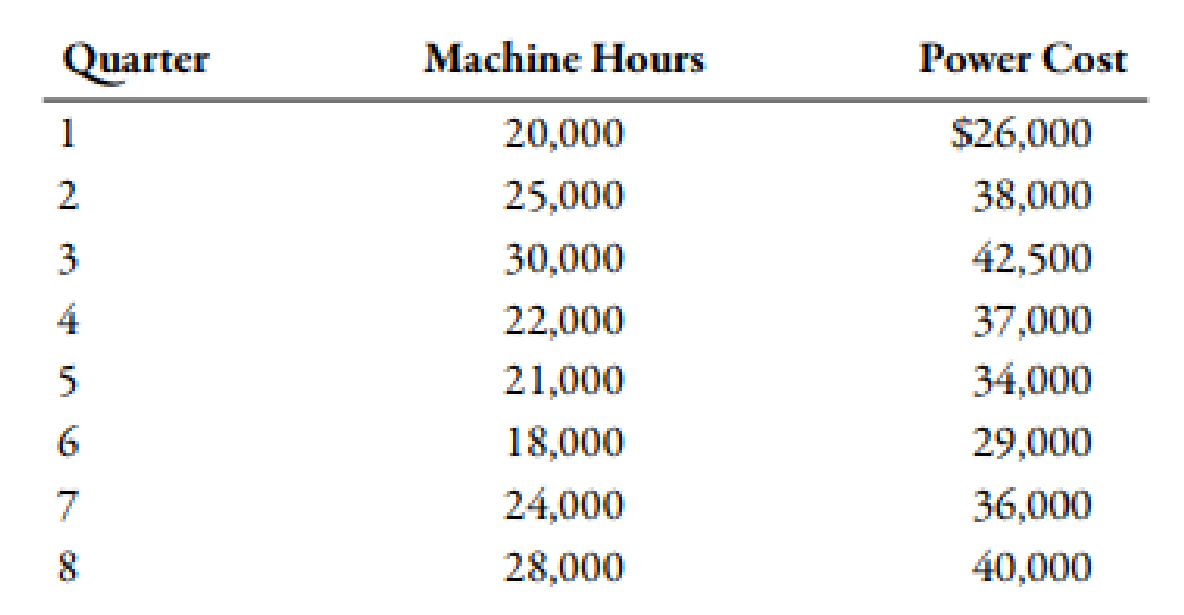Chapter 3, Problem 68P### Managerial Accounting: The Corners...

7th Edition
Maryanne M. Mowen + 2 others
ISBN: 9781337115773

#### Solutions

Chapter
Section### Managerial Accounting: The Corners...

7th Edition
Maryanne M. Mowen + 2 others
ISBN: 9781337115773
Textbook Problem
168 views

# (Appendix 3A) Scattergraph, High-Low Method, Method of Least Squares, Use of JudgmentThe management of Wheeler Company has decided to develop cost formulas for its major overhead activities. Wheeler uses a highly automated manufacturing process, and power costs are a significant manufacturing cost. Cost analysts have decided that power costs are mixed. The costs must be broken into their fixed and variable elements so that the cost behavior of the power usage activity can be properly described. Machine hours have been selected as the activity driver for power costs. The following data for the past 8 quarters have been collected:Note: For the following requirements, round the fixed cost to the nearest dollar, round the variable rates to three decimal places, and the R2 to two decimal places.Required: 1. Prepare a scattergraph by plotting power costs against machine hours. Does the scatter-graph show a linear relationship between machine hours and power cost? 2. Using the high and low points (i.e., the high-low method), compute a power cost formula. (Note: Round answers to three decimal places.) 3. Use the method of least squares to compute a power cost formula. Evaluate the coefficient of determination. 4. CONCEPTUAL CONNECTION Rerun the regression, and drop the point (20,000, \$26,000) as an outlier. Compare the results from this regression to those for the regression in Requirement 3. Which is better?

1.

To determine

Construct the scatter graph by plotting the costs of power and machine hours. Also, identify the relationship between the costs of power and machine hours.

Explanation

Scatter Graph Method:

In scatter graph method, data points are plotted on the graph to visualize the relationship between total cost and output measure.

Scatter graph of cost of power and machine hours:

2.

To determine

Construct the power cost formula with the help of high-low method.

3.

To determine

Construct the cost formula with the help of least square. Also, evaluate the result.

4.

To determine

Compare the results of R3 with the R2.

### Still sussing out bartleby?

Check out a sample textbook solution.

See a sample solution

#### The Solution to Your Study Problems

Bartleby provides explanations to thousands of textbook problems written by our experts, many with advanced degrees!

Get Started